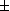# Graphic abs --- Introduction ---

Graphic abs is an exercise concerning the graphical recognition of functions of one real variable involving absolute values.

The server will give you the graph of a function f (x), whose expression will be hidden. You will then be presented a certain number of other graphs, corresponding to modifications of f (x) such as f (|x|), |f (x)|, or even |f (x)|+1, etc (see the menu below). And you should cocate the one which corresponds to one which will be precised in a question. To give the reply, you can simply click on the graph which you believe to be the good one.

The exercise can be configured by the following parameters.
• Type of variations of the function:
1. f (|x|), |f (x)| ;
2.f (|x|), |f (x)| ;
3.|f (x)|, f (|x|) ;
4.|f (x)|,f (|x|) ;
5. f (|x|), |f (x)|, f (|x|)1, |f (x)|1, |f (x)1|.
• Numbre of questions in one session: , , , , , , , (The score is attributed only at the end of a session.)
• or you can be asked to recognize each presented curve.

Other exercises on: graphic   absolute value   Functions

In order to access WIMS services, you need a browser supporting forms. In order to test the browser you are using, please type the word wims here: and press Enter''.